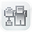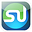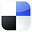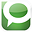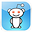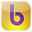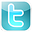Excel
STATISTICAL
Master

Correlation and Covariance

Clear and Complete - WITH LOTS OF SOLVED PROBLEMS

# Correlation and Covariance

Lots of Worked-Out, Easy-To-Understand, Graduate-Level Problems  --->
( Scroll Down and Take a Look ! )

Introduction

Correlation and Covariance describe linear relationships between different variables. Both Correlation and Covariance describe whether variables move together in the same direction, move in opposite directions, or don't move in any
related way at all. Correlation and Covariance also describe how strong these linear relationships are between the variables.

## Basic Explanation of Correlation and Covariance

Correlation and Covariance are very similar ways of describing the direction and strength of linear relationships between two variables. Correlation is a more well-known concept and more widely used. It will therefore be covered in the first half of this course module. Covariance will be covered in the second half.

Correlation Analysis

Positive Correlation vs. Negative Correlation

Positive Correlation

If two variables are "positively correlated," they move in the same direction. When one goes up, the other goes up as well. Two variables that are positively correlated have a correlation coefficient that is between 0 and +1. The closer the correlation coefficient is to +1, the more exactly the two variables move together.  A correlation coefficient between two variables of exactly +1.00 means that both variables move in lock-step with each other. A correlation coefficient between two variable of 0 indicates that there is no relationship between the movement of one variable and movement of the other variable.

Negative Correlation

If two variables are "negatively correlated," they move in opposite directions. When one goes up, the other goes down. When one variable goes down, the other goes up. Two variables that are "negatively correlated" have a correlation coefficient that is between -1 and 0. The closer the correlation coefficient is to -1, the more exactly the two variables move in opposite directions. A correlation coefficient between two variables of exactly -1.00 means that both variables move lock-step with each other in opposite directions. A correlation coefficient between two variable of 0 indicates that there is no relationship between the movement of one variable and movement of the other variable.

A general way to interpret the calculated r value is as follows:

0.0 to 0.2  -  Very weak to negligible correlation
0.2 to 0.4  -  Weak correlation
0.4 to 0.7  -  Moderate correlation
0.7 to 0.9  -  Strong correlation
0.9 to 1.0  -  Very strong correlation

Calculation of Correlation Coefficient

The correlation also describes how linear a relationship is between two variables. The Correlation Coefficient can have values between -1 and +1. Below is the formula for calculating the Correlation Coefficient. Excel does such a great job in calculating correlation and covariance that it is not necessary to memorize the formulas of covariance and correlation, if you have access to Excel and know how to use the correlation functions. Here are the correlation formulas below:

***********************************************************

Population Correlation Coefficient

Correlation of variables x and y from a known population = ρ ("rho")

ρ = Population Correlation Coefficient

ρ
= ( Covariance of x and y) / ( Standard Deviation of x * Standard Deviation of y )

ρ  = σxy / ( σx * σy)

(Covariance will be explained later in this module)

***********************************************************

Sample Correlation Coefficient

Correlation of variables x and y randomly sampled from an unknown population = r      (This is the normal situation)

r = Sample Correlation Coefficient

The Sample Correlation Coefficient, r, is also known as the Product Moment Coefficient or Pearson's Correlation.

r = ( Sample Covariance of x and y) / ( Sample Standard Deviation of x * Sample Standard Deviation of y )

r = Sample Correlation Coefficient = Sxy / (Sx * Sy)

rxy = [ nΣxiyi - ΣxiΣyi ]  /   [ SQRT( nΣxi2 - (Σxi)2 ) * SQRT(nΣyi2 - (Σyi)2)]

Correlation does not mean causation. Correlation indicates that there might be causation between two variables but this may not be the case at all. There might be underlying causes there are not known and the correlation is merely incidental. Variables that provide causation but are not included in the correlation are often called "confounding variables."

The Pearson correlation, r, indicates the strength of a linear relationship between variables. There might also be non-linear relationships between variables. Visual examination of a graphing of the data points can sometimes show a non-linear relationship between the variables that would not be evident from the correlation analysis. It is always important to look at the data points on a graph in addition to any numerical analysis performed on the data.

Problem 1

Problem 1: Calculating Correlation Between Two Variables

Problem: Calculate the correlation between variables x and y based on the 6 pairs of x-y data given below:

 x y 1 2 3 6 6 7 8 9 5 6 4 5

The Correlation of variables x and y is rxy:

Using the above formula:

r = Sample Correlation Coefficient = Sxy / (Sx * Sy)

rxy = [ nΣxiyi - ΣxiΣyi ]  /   [ SQRT( nΣxi2 - (Σxi)2 ) * SQRT(nΣyi2 - (Σyi)2)]

The data needs to be arranged in following way to facilitate correlation analysis:

 X Y XY X2 Y2 1 2 2 1 4 3 6 18 9 36 6 7 42 36 49 8 9 72 64 81 5 6 30 25 36 4 5 20 16 25 Totals 27 35 184 151 231 ΣX ΣY ΣXY ΣX2 ΣY2

Number of samples = n = 6

Xavg = ΣX / n = 27 / 6 = 4.5

Yavg = ΣY / n = 35 / 6 = 5.83

rxy = Correlation Coefficient between X and Y

rxy = [ n* ΣXY – ΣX*ΣY ] / [ SQRT(n*ΣX2 – (ΣX)2) * SQRT ( n*Σy2 – (Σy)2 ) ]

rxy = [ 6*184 – 27*35 ] / [ SQRT(6*151 – (27)2) * SQRT ( 6* 231 – (35)2 ) ]

rxy = 0.94

This same problem above is solved in the Excel Statistical Master with only 1 Excel formula. If you found your statistics book confusing, You'll really like the Excel Statistical Master. Everything is explained in simple, step-by-step frameworks.

Problem 2

Problem 2: Calculating Correlation Between Multiple Variables

Problem: Determine the correlation between all of the variables below:

 x y z a 1 2 10 24 3 6 9 45 6 7 8 56 8 9 7 46 5 6 6 67 4 5 5 23

We assume this data is sample data so the Correlation Coefficient is r, not σ which is the Correlation Coefficient for data from a known population.

Using the above formula:

rxy = [ nΣxiyi - ΣxiΣyi ]  /   [ SQRT( nΣxi2 - (Σxi)2 ) * SQRT(nΣyi2 - (Σyi)2)]

The correlation between x and y = rxy =  0.94

The correlation between x and z = rxz = -0.51

The correlation between x and a = rxa =  0.55

The correlation between y and z = ryz = - 0.39

The correlation between y and a = rya =   0.59

The correlation between z and a = rza = - 0.16

This same problem aboveis solved in the Excel Statistical Master with only 1 Excel formula. The Excel Statistical Master is the fastest way for you to climb the business statistics learning curve.

The closer to +1 the correlation between 2 variables is, the more they move together in the same direction.

The closer to -1 the correlation between 2 variables is, the more they move in opposite directions.

The closer to 0 the correlation between 2 variables is, the less related and more random is their movement.

r2 - Square of Correlation Coefficient

r2 - The square of the correlation coefficient, known as the Sample Coefficient of Determination, calculates what percentage of total variance of the output (dependent variable) of a regression is explained by the variance of the inputs (independent variables). In other words, r Square represents the proportion of the total variation that is explained by the regression equation.

Total Variance = Explained Variance + Unexplained Variance

Σ (Y – Yavg)2       = Σ (Yest – Yavg)2         + Σ (Y – Yest)2

r Square = Sample Coefficient of Determination

r Square = Explained Variance / Total Variance

Since Total Variance = Explained Variance + Unexplained Variance

Σ (Y – Yavg)2                 = Σ (Yest – Yavg)2           + Σ (Y – Yest)2

r Square = Explained Variance / Total Variance

r Square = 1 - [ Unexplained Variance / Total Variance ]

r Square = 1 - [ Σ (Y – Yest)2 / Σ(Y – Yavg)2 ]

## Covariance Analysis

The covariance also describes how linear a relationship is between two variables.

The main difference between covariance and correlation is the range of values  that each can assume. The Correlation between two variables can assume values only between -1 and +1. The Covariance between two variables can assume a value outside of this range. The more positive a covariance is, the more closely the variables move in the same direction. Conversely, the more negative a covariance is, the more the variables move in opposite directions.

Two independent variables will have a zero Covariance. A Covariance of zero does not that two variables are independent though. The two variables may have a nonlinear relationship. This may not be picked up at all by the Covariance calculation.

The Covariance calculation between two variables is very dependent upon the scale that the two variables are measured by. This is the main disadvantage of using Covariance instead of Correlation to compare two variables. The Correlation Coefficient is not dependent upon the scale used and provides the ability to compare different sets of data consistently.

Calculation of Covariance

Below is the formula for calculating the Covariance of variables. Excel does such a great job in calculating correlation and covariance that it is not necessary to memorize the formulas of covariance and correlation, but here they are, along with examples worked out in Excel:

Covariance of variables x and y from a known population = σxy

σxy = 1/n * Σ (xi - µx) * (yi - µy) as i goes from 1 to n

µx and µy represent population means and sxy represents a covariance from a population.

Covariance of variables x and y randomly sampled from an unknown population = sxy    (This is the normal situation)

sxy = 1/ (n - 1) * Σ (xi - xavg) * (yi - yavg) as i goes from 1 to n

Problem 3

Problem 3: Calculating Covariance Between Two Variables

Problem: Calculate the covariance between variables x and y based upon the 6 pairs of x-y data given below:

 x y 1 2 3 6 6 7 8 9 5 6 4 5

Using the above formula:

sxy = 1/ (n - 1) * Σ (xi - xavg) * (yi - yavg) as i goes from 1 to n

The Covariance of variables x and y is sxy:

sxy = 4.42

This same problem above is solved in the Excel Statistical Master with only 1 Excel formula. With the Excel Statistical Master you can do advanced business statistics without having to buy and learn expensive, complicated statistical software packages such as SyStat, MiniTab, SPSS, or SAS.

Problem 4

Problem 4: Calculating Covariance Between Multiple Variables

Problem: Determine the covariance between all of the variables below:

 x y z a 1 2 10 24 3 6 9 45 6 7 8 56 8 9 7 46 5 6 6 67 4 5 5 23

We assume this data is sample data so the Covariance variable is sxy, not σxy which is the Covariance variable for data from a known population.

Using the above formula:

sxy = 1/ (n - 1) * Σ (xi - xavg) * (yi - yavg) as i goes from 1 to n

The covariance between x and y = sxy = 4.42

The covariance between x and z = sxz = -1.92

The covariance between x and a = sxa = 19.25

The covariance between y and z = syz = -1.42

The covariance between y and a = sya = 19.75

The covariance between z and a = sza = -4.25

This same problem above is solved in the Excel Statistical Master with only 1 Excel formula. The Excel Statistical Master is the fastest way for you to climb the business statistics learning curve.

The more positive the covariance between 2 variables is, the more they move together in the same direction.

The more negative the covariance between 2 variables is, the more they move in opposite directions.

Variance Relationships Derived From Covariance

Variance of a Sum = (σx+y)2 = σx2 + σy2 + 2Covxy

Variance of a Difference = (σx-y)2 = σx2 + σy2 - 2Covxy

Variance of a Variable + a Constant = (σx+C)2 = σx2

Variance of a Variable - a Constant = (σx-C)2 = σx2

Variance of a Variable times a Constant = (σCx)2 = σx2 * C2

Variance of a Variable divided by a Constant = (σx/C)2 = σx2 / C2

If You Like This, Then Share It...